# Resistance, Resistivity & Sheet Resistance

Back to Posts

You’ll often hear the terms resistance, resistivity, conductivity, sheet resistance, etc. thrown about. These are all related, and are different ways of answering this question:

How easy is it for electricity to move through the material?

There’s a detailed explanation here, but it’s pretty boring. Read on for the slightly-less-boring version.

## Resistance - R (Ω)

### What is it?

Resistance (R), measured in Ohms (Ω), tells you how much effort it takes to move electricity through an object — or, phrased differently, it tells you how much an object resists the flow of current.

### How do we use it?

Primarily in Ohm’s Law, the workhorse of basic electronics calculations: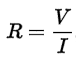If you know the resistance of a thing, you can get current at some applied voltage, or vice-versa.

### How do we talk about it?

Resistance is specific to a single object.

### GOOD:

“What is the resistance of this trace?”

“What is the resistance of this piece of wire?”

“What is the resistance of copper?”

Why bad: This doesn’t mean anything. For this, you need resistivity!

## Resistivity - ρ (Ω.m)

### What is it?

Resistivity (ρ) is the fundamental property of a bulk material that tells you how much resistance a thing made of that material will have, if you know its shape (length, width, height). (and temperature… but let’s not worry about that.)

Why is this useful? Let’s say I have to design two wires to have identical resistance — one is made of copper, the other one is a Twizzler. If I try to force electricity through both, I’m sure you can guess that the one made of copper will allow electricity to flow much easier. The Twizzler will not: it will just get electrically cooked from the inside, which is actually a food truck idea I’ve been working on. Knowing the resistivity will let me adjust the length of the wire properly to match their resistances. We’re gonna need quite a bit of copper.

### How do we use it?

You’ll use resistivity if you’re trying to find out the resistance of an object made of some material. For a wire, the standard equation is: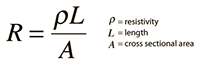If you know the length and cross-section area, you get our old friend resistance.

### How do we talk about it?

Resistivity is specific to a type of material.

### GOOD:

“What is the resistivity of the conductive ink?”

“What is the resistivity of copper?”

“What is the resistivity of this trace?”

Why bad: At worst, meaningless. At best, ambiguous. If someone asks this, assume that they’re asking for the material it’s made of and answer as such — but with an air of superiority.

“What is the resistivity of the circuit?”

Why bad: You should feel bad. You just put together two words that sound related. Your ancestors are watching, crying hot tears of shame.

## Sheet Resistance - Rs (Ω/sq)

### What is it?

Resistance and Resistivity are both pristine, beautiful science words. Sheet Resistance (Rs) is a dirty, uncultured engineering word — it exists only to make calculations easier, because thinking too hard may kill an engineer. Engineers are notorious for their shortcuts, because all they care about is lame stuff like “accomplishing things” or “making tangible progress.” Not us scientists. Anyways, sheet resistance has units of Ohms per square.

I know, square isn’t a unit — square is a shape. Tell that to engineers.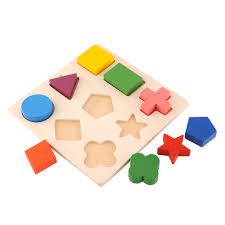Engineering exam question — which of these is a unit?

Anyway, Sheet Resistance is a combination of resistivity of a material and its shape, and is a shortcut for calculating resistance when designing PCBs.

### How do we use it?

If you have the sheet resistance Rs, all you need is the ratio of length (L) to width (W) to find the resistance, like so: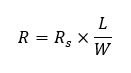Why is this easier? Well, in PCB design you usually already know the thickness (t) of the material (35um or 70um) and type of material (annealed copper), so you know sheet resistance. All you need is to measure the length and width of a trace, and there you have it — resistance!

Side note — you can also easily convert from sheet resistance to resistivity: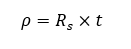### How do we talk about it?

Typically you’d reference sheet resistance in terms of a material with a thickness.

### GOOD:

“The sheet resistance of 1 oz copper is 0.5 mOhm/sq.”

“The sheet resistance of conductive ink at 70um thickness is 12 mOhm/sq.”

“Square is a legitimate unit.”

If resistance tells you how hard it is to push electricity through a material, conductance tells you how easy it is to push electricity through a material.

“Wait a minute…” you sputter. “That’s the same thing!”

Yup.

Conductance (G), in units of Siemens (S) is just the reciprocal of resistance (R).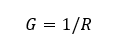Likewise, conductivity (σ), in units of Siemens per meter (S/m), is the reciprocal of resistivity (ρ).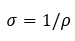So if someone asks for conductivity, they’re really asking you for resistivity in disguise.Now you know the impossible truth.

## Did I heard someone say the word “impedance”?

Woah, slow down bronco. Impedance is a bit more complicated — for now just think of impedance as resistance, but when you’re dealing with things that aren’t resistors — things that require a different amount of effort to push a signal through the wire because of the frequency of the signal.

TLDR: Impedance (Z) is like resistance, but mainly focuses on making students cry. When circuits have signals that operate at a certain frequency (ex: a clock signal that turns LEDs on at set intervals), that signal may flow more easily or difficultly depending on the frequency. Impedance is a complex number where resistance (R) is the real part and reactance (X) is the imaginary part. Clearly this requires a separate blog post, because since it deals with the imaginary part of impedance, it will be an imaginary blog post.

## In conclusion

To sum it up: resistance is a property of a given object, resistivity is a property of a given material and sheet resistance is a filthy-but-useful engineering shortcut that treats shape as a unit. Your ancestors can finally enjoy their well-earned forever sleep.